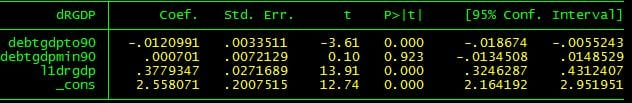## Debt and Growth III

I’m going to try to make this post brief and comprehensible.  It contains no information not in an earlier post but I delete a whole lot of distracting data.

The question is does the Reinhart Rogoff (hence R-R) data set on public debt and real GDP in 20 rich countries post WWII contain evidence that a debt to gdp ratio higher than 90% causes lower real GDP growth.  My answer is no it does not contain any such evidence.

Note the very very low bar.  I claim no evidence of a bad effect not just no evidence that 90% is a critical level at which additional debt becomes extremely bad for growth but no evidence of any effect on growth at all.

Why is my result different from almost everyon else’s (except for Dube’s) ?  Well I take the very very first step to address causation — I run a regression including lagged real GDP growth.  This is the first standard totally unconvincing way to try to determine causation from historical data.  A significant estimated effect of debt would mean that debt “Granger causes” low growth where Granger cause is the English translation of post hoc ergo propter hoc.the dependent variable is annual real GDP growth.  The first coefficient is on the debt to gdp ratio in percent rounded down to 90 if it is over 90.  The second coefficient — the coefficient of interest — is the ratio minus 90 if it is over 90 or zero if it is under 90.  This coefficient is an estimate of the effect of further debt once debt has already reached 90% of GDP — exactly the effect of interest.  l1drgdp is the one year lagged rate of real GDP growth. _cons is aconstant term.

The point is that the coefficient on debtgdpmin90 is actually very slightly positive.  There is no evidence in the R-R data set that debt to GDP ratios greater than 90% are worse for growth than a debt ratio of 90%.

Here is the stata do file which generates the result.  It uses the R-R data set as processed a bit by Herndon et al and available here

clear
use C:\rjw\Papers\Peri\RR-processed.dta

quietly tab Country,gen(count)
gen cntry = count1+2*count2+3*count3+4*count4+5*count5+6*count6 + 7*count7 + 8*count8+9*count9+10*count10+11*count11+12*count12+13*count13+14*count14+15*count15+16*count16+17*count17+18*count18+19*count19+20*count20

gen l1drgdp = dRGDP[_n-1] if cntry[_n-1]==cntry

gen debtgdpto90 = debtgdp + (90-debtgdp)*(debtgdp>90)
gen debtgdpmin90 = debtgdp-debtgdpto90

gen debtgdpto30 = debtgdp + (30-debtgdp)*(debtgdp>30)
gen debtgdpmin30 = debtgdp-debtgdpto30

reg dRGDP debtgdpto90 debtgdpmin90 l1drgdp

Tags: ,